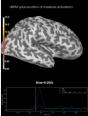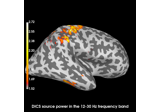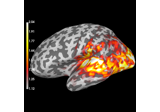# mne.viz.plot_cov#

mne.viz.plot_cov(cov, info, exclude=(), colorbar=True, proj=False, show_svd=True, show=True, verbose=None)[source]#

Plot Covariance data.

Parameters:
covinstance of `Covariance`

The covariance matrix.

info`mne.Info`

The `mne.Info` object with information about the sensors and methods of measurement.

exclude

List of channels to exclude. If empty do not exclude any channel. If ‘bads’, exclude info[‘bads’].

colorbarbool

Show colorbar or not.

projbool

Apply projections or not.

show_svdbool

Plot also singular values of the noise covariance for each sensor type. We show square roots ie. standard deviations.

showbool

Show figure if True.

verbose

Control verbosity of the logging output. If `None`, use the default verbosity level. See the logging documentation and `mne.verbose()` for details. Should only be passed as a keyword argument.

Returns:
fig_covinstance of `matplotlib.figure.Figure`

The covariance plot.

fig_svdinstance of `matplotlib.figure.Figure` | `None`

The SVD spectra plot of the covariance.

Notes

For each channel type, the rank is estimated using `mne.compute_rank()`.

Changed in version 0.19: Approximate ranks for each channel type are shown with red dashed lines.

## Examples using `mne.viz.plot_cov`#Source localization with MNE, dSPM, sLORETA, and eLORETA

Source localization with MNE, dSPM, sLORETA, and eLORETACompute evoked ERS source power using DICS, LCMV beamformer, and dSPM

Compute evoked ERS source power using DICS, LCMV beamformer, and dSPMCompute source power estimate by projecting the covariance with MNE

Compute source power estimate by projecting the covariance with MNE